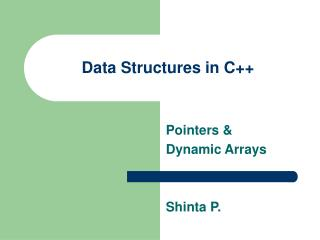DownloadDownload PresentationData Structures in C++

# Data Structures in C++

Download Presentation## Data Structures in C++

- - - - - - - - - - - - - - - - - - - - - - - - - - - E N D - - - - - - - - - - - - - - - - - - - - - - - - - - -
##### Presentation Transcript

1. Data Structures in C++ Pointers & Dynamic Arrays Shinta P.

2. Static and Dynamic Memory • Static Allocation • allocated by the compiler at compile time • once allocated, does not change • Dynamic Allocation • allocated by program at run time • ‘new’ allocates desired amount from heap • amount depends on class/type • ‘delete’ deallocates an object and returns to storage manager for reallocation

3. Where or When • Static • data stricture sizes are fixed • little chance for storage needs to grow • prototypes • Dynamic • amount of data changes from run to run • data relationships change frequently

4. Pointers • a built-in primitive type; 32 bit • used to hold the storage address of a variable • to define a pointer variable • use the * operator in the definition eg. int *airplane_ptr ; (airplane_ptr is a variable that will point to an integer)

5. Pointers (cont.) • To assign a value to the pointer variable • use the address operator & eg. int F15; int Airplane_ptr; Airplane_ptr = &F15; Airplane_ptr F15

6. Pointers (cont.) • Note that F15 has not been assigned a value yet • to do this we must use the dereferencing operator * ex. *Airplane_ptr = 5 (dereferencing * reads: location pointed to by var) 5 Airplane_ptr F15

7. Pointers (cont.) • Which is exactly equivalent to: F15 = 5; ...so whats the big deal??

8. The Big Deal.... • We’ve been looking at the trivial case • Pointers to primitives aren’t very useful • things get more interesting with arrays • we can make : • an array that grows as the application needs more room for data • an array that shrinks as the application needs less room for data • and much better with dynamic objects

9. More Pointers int i = 50; int j = 75; int *p1 ; int * p2 p1 = &i ; p2 = & j; cout << *p1; p1 = p2 ; *p2 =0; cout <<*p1;

10. Pointers to arrays • The name of an array is a pointer to the 0th element of the array (the beginning of the array) int array ; // array is equivalent to & array *array = 5; is like array = 5; int j = *(array+4) is like int j = array cout << *array; is like cout << array;

11. Pointers to arrays • Pass by reference - in C++ arrays are always pass by reference (there is no pass by value for an array) • this is a big improvement over C • in C to pass an array to a function it had to be passed by passing a pointer to the beginning of the array then doing pointer arithmetic to manipulate the contentsd of the array

12. new • returns the address of a piece of dynamically allocated storage ex. int *i; //create a pointer i = new int // get a new integer *i = 75 // assign it a value 75 i

13. Dynamic Arrays • arrays can be allocated at run time double * p; int count ; cout << “how many elements? “ << “\n”; cin >> count; p = new double[count];

14. Dynamic Arrays • You can effectively change the size of an array at run-time if it was originally allocated dynamically. … from previous example double * temp; temp = new double; /* copy the contents of the old array into the new one */ for (int I=0 ; I < 10 ; I++) temp[I] = p[I]; /* dispose of the original array */ delete p; p = temp; /* now the array has twice as many elements */

15. Value semantics • The value semantics of a class determine how values are copied from one object to another. • In C++ the value semantics consist of two operations: • the assignment operator • the copy constructor • The copy constructor is a constructor that creates and initializes an object to the value of another (existing) object • the copy constructor has one parameter whose type is the same as the class name Ex. Date Today; Today.month=5 ; Today.year=2000; Today.day = 21; Date Tomorrow(Today)

16. Copy Constructor Date :: Date(const & Date t) { month = t.month; day = t.month; year = t.year; }